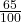Question

a phone is 35 percent off which is now worth 78 pounds what was the original price

1.120 pounds

Step-by-step explanation:

65% of y= 78 pounds× y = 78 pounds

65y = 78  × 100=7800

y= 7800/65= 120 pounds